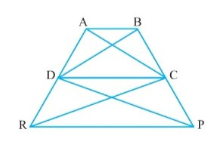Q

# In Fig.9.29, ar (DRC) = ar (DPC) and ar (BDP) = ar (ARC). Show that both the quadrilaterals ABCD and DCPR are trapeziums.

Q : 16    In Fig.,    and . Show that both the quadrilaterals ABCD and DCPR are trapeziums.ViewsGiven,
ar(DPC) = ar(DRC) ..........(i)
and ar(BDP) = ar(ARC)............(ii)

from equation (i),
Since DRC and DPC lie on the same base DC and between same parallels.
CD || RP (opposites sides are parallel)

Hence quadrilateral DCPR is a trapezium

Now, by subtracting eq(ii) - eq(i) we get

ar(BDP) - ar(DPC) = ar(ARC) - ar(DRC)
ar(BDC) = ar(ADC) (Since theya are on the same base DC)
AB || DC

Hence ABCD is a trapezium.

Exams
Articles
Questions